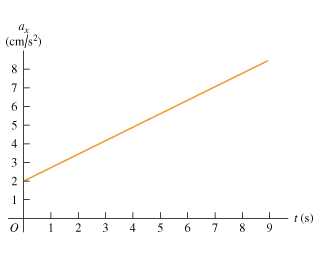# Problem: The graph in the figure describes the acceleration as a function of time for a stone rolling down a hill starting from rest. (a) Find the change in the stones velocity between t = 2.5 and t = 7.5. (b) Sketch a graph of the stones velocity as a function of time.

###### FREE Expert Solution
94% (206 ratings)
###### Problem Details

The graph in the figure describes the acceleration as a function of time for a stone rolling down a hill starting from rest. (a) Find the change in the stones velocity between t = 2.5 and t = 7.5. (b) Sketch a graph of the stones velocity as a function of time.